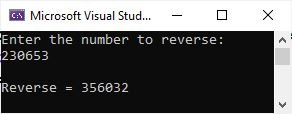# C# program to reverse a number

This post was published to show our users how we can reverse a number using a C# program. For example, if the number is 236, its reverse should be 632.

## Simple C# program to reverse a number

Let me first create a simple program in C# that reverses a number, say 2945.

```int num = 2945;
int rem, rev = 0;

while(num != 0)
{
rem = num % 10;
rev = rem + (rev * 10);
num = num / 10;
}

Console.WriteLine("Reverse = " +rev);```

This C# program that shows the reverse of a number should print out as follows:

`Reverse = 5492`

## C# program to reverse a number entered by the user

Now let me create another C# program that does the same job as the previous one, but this program allows the user to enter the number.

```int num, rem, rev = 0;

Console.WriteLine("Enter the number to reverse: ");

while(num != 0)
{
rem = num % 10;
rev = rem + (rev * 10);
num = num / 10;
}

Console.WriteLine("\nReverse = " +rev);```

With user input 230653, the output produced by this C# example is shown in the snapshot given below, which was taken when I was executing this program in the Microsoft Visual Studio IDE.C# Online Test

« Previous Program Next Program »